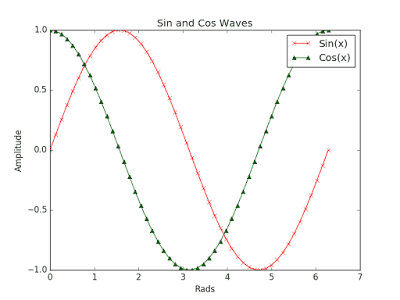# 十分钟入门MatplotlibPython 在处理数据、分析数据以及数据可视化方面拥有很多功能强大的工具，这也是 Python 在科学领域中能够迅速发展的一个主要原因。

## 画一个简单的图形

```import matplotlib.pyplot as plt
import numpy as np
```

```# 简单的绘图
x = np.linspace(0, 2 * np.pi, 50)
plt.plot(x, np.sin(x)) # 如果没有第一个参数 x，图形的 x 坐标默认为数组的索引
plt.show() # 显示图形
```

`plot` 命令以一种简洁优雅的方式创建了图形。提醒一下，如果没有第一个参数 x，图形的 x 轴坐标将不再是 0 到 2pi，而应该是数组的索引范围。## 在一张图上绘制两个数据集

```x = np.linspace(0, 2 * np.pi, 50)
plt.plot(x, np.sin(x),
x, np.sin(2 * x))
plt.show()
```## 自定义图形的外观

```# 自定义曲线的外观
x = np.linspace(0, 2 * np.pi, 50)
plt.plot(x, np.sin(x), 'r-o',
x, np.cos(x), 'g--')
plt.show()
```## 使用子图

```# 使用子图
x = np.linspace(0, 2 * np.pi, 50)
plt.subplot(2, 1, 1) # （行，列，活跃区）
plt.plot(x, np.sin(x), 'r')
plt.subplot(2, 1, 2)
plt.plot(x, np.cos(x), 'g')
plt.show()
```## 简单的散点图

```# 简单的散点图
x = np.linspace(0, 2 * np.pi, 50)
y = np.sin(x)
plt.scatter(x,y)
plt.show()
```## 彩色映射散点图

```# 彩色映射散点图
x = np.random.rand(1000)
y = np.random.rand(1000)
size = np.random.rand(1000) * 50
colour = np.random.rand(1000)
plt.scatter(x, y, size, colour)
plt.colorbar()
plt.show()
```## 直方图

```# 直方图
x = np.random.randn(1000)
plt.hist(x, 50)
plt.show()
```## 标题，标签和图例

```# 添加标题，坐标轴标记和图例
x = np.linspace(0, 2 * np.pi, 50)
plt.plot(x, np.sin(x), 'r-x', label='Sin(x)')
plt.plot(x, np.cos(x), 'g-^', label='Cos(x)')
plt.legend() # 展示图例
plt.ylabel('Amplitude') # 给 y 轴添加标签
plt.title('Sin and Cos Waves') # 添加图形标题
plt.show()
```### 不要忘记分享和关注

Python 翻译组是EarlGrey@编程派发起成立的一个专注于 Python 技术内容翻译的小组，目前已有近 30 名 Python 技术爱好者加入。翻译组出品的内容（包括教程、文档、书籍、视频）将在编程派微信公众号首发，欢迎各位 Python 爱好者推荐相关线索。推荐线索，可直接在编程派微信公众号推文下留言即可。